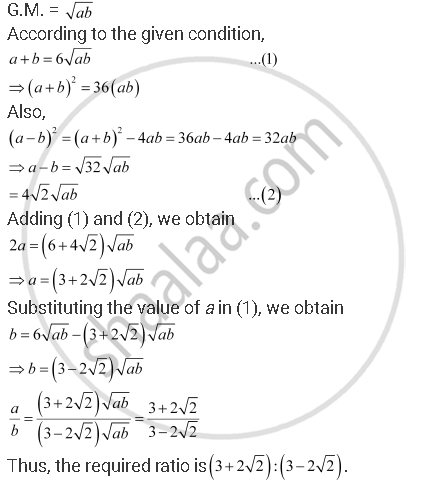CBSE (Arts) Class 11CBSE
Share
Notifications

View all notifications
Books Shortlist
Your shortlist is empty

# The Sum of Two Numbers is 6 Times Their Geometric Mean, Show that Numbers Are in the Ratio 3 + 2sqrt2) ":" (3 - 2sqrt2) - CBSE (Arts) Class 11 - Mathematics

Login
Create free account

Forgot password?
ConceptGeometric Progression (G. P.)

#### Question

The sum of two numbers is 6 times their geometric mean, show that numbers are in the ratio 3 + 2sqrt2) ":" (3 - 2sqrt2)

#### Solution

Let the two numbers be a and b.Is there an error in this question or solution?

#### APPEARS IN

NCERT Solution for Mathematics Textbook for Class 11 (2018 to Current)
Chapter 9: Sequences and Series
Q: 28 | Page no. 193
Solution The Sum of Two Numbers is 6 Times Their Geometric Mean, Show that Numbers Are in the Ratio 3 + 2sqrt2) ":" (3 - 2sqrt2) Concept: Geometric Progression (G. P.).
S